Andrea Morgan

•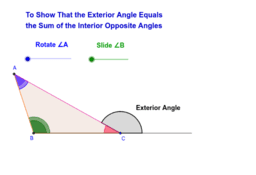The relationship between the exterior and interior angles of a triangle.

Activity

Andrea Morgan

•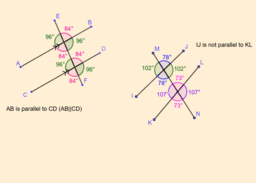Parallel lines and angles

Activity

Andrea Morgan

•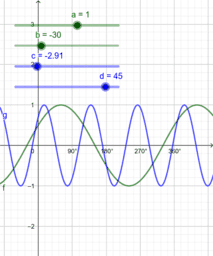Equating Cos and Sin Trig functions

Activity

Andrea Morgan

•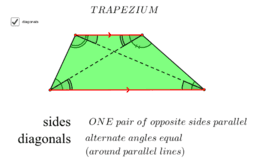Properties of a Trapezium

Activity

Andrea Morgan

•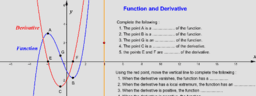2. A cubic function and its derivatives

Activity

Andrea Morgan

•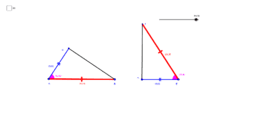Congruence of Triangles

Book

Andrea Morgan

•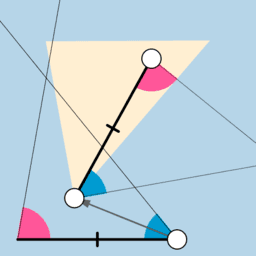Congruency: ASA

Activity

Andrea Morgan

•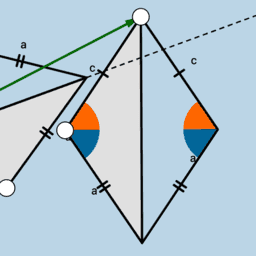Congruency: SSS

Activity

Andrea Morgan

•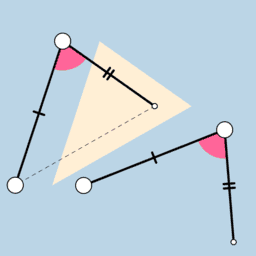Congruency: SAS

Activity

Andrea Morgan

•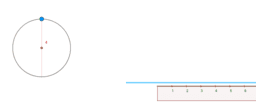Investigating the Circumference of a Circle and Pi

Activity

Andrea Morgan

•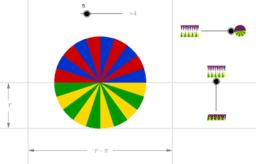The Area of a circle

Activity

Andrea Morgan

•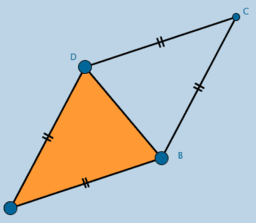Rhombus

Activity

Andrea Morgan

•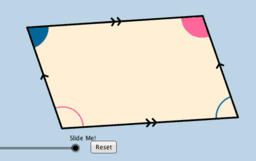Parallelograms

Activity

Andrea Morgan

•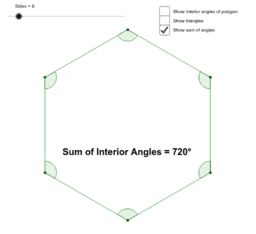Sum of the Interior Angles of a Regular Polygon

Activity

Andrea Morgan

•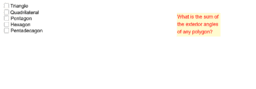The Sum of Exterior Angles of Polygons

Activity

Andrea Morgan

•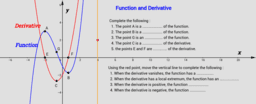2. A cubic function and its derivatives

Activity

Andrea Morgan

•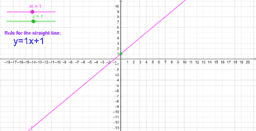Introduction to straight line graphs

Activity

Andrea Morgan

•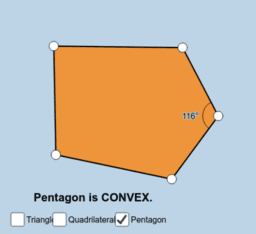Convex Pentagon and the effect of the change in the size of one interior angle

Activity

Andrea Morgan

•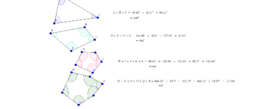Interior Angles of different Polygons

Activity

Andrea Morgan

•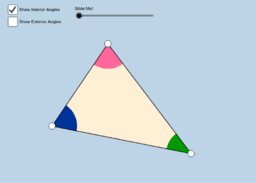Interior angles and the sum of exterior angles

Activity

Andrea Morgan

•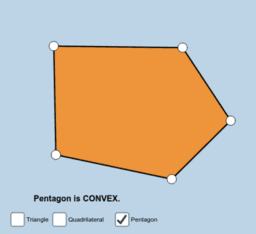Convex and concave polygons

Activity

Andrea Morgan

•Regular polygons

Activity

Andrea Morgan

•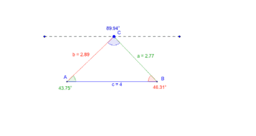Relationship between lengths of sides and opposite angles in triangles

Activity

Andrea Morgan

•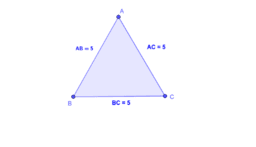Classifying triangles

Activity

Andrea Morgan